13 篇文章 62 订阅

一、从BPSK调制解调器说起

BPSK是二进制相移键控，是最基本的调制方式。本科时有一个经典的实验便是比较不同调制方式下的误码率曲线，并且与理论误码率曲线进行作比较。其实要完成这个实验，网上随随便便都可以查到理论的仿真代码，不需要信道编码、捕获、同步、匹配滤波、多普勒频偏等考量，但是一个仿真要越接近实际系统，便需要考虑的因素越多，代码难度便越大。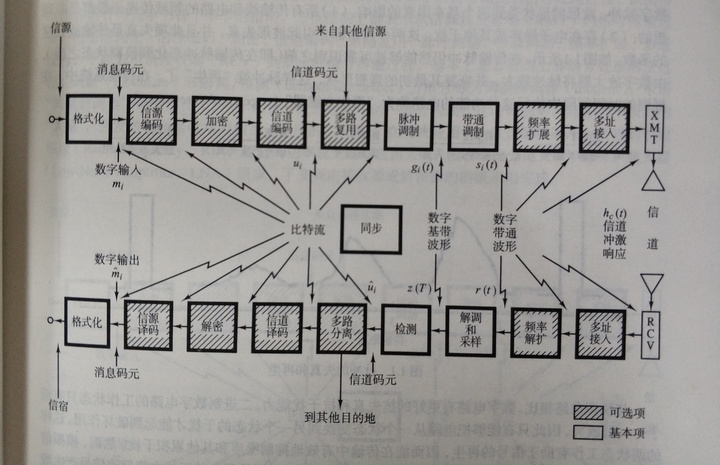二、代码规范

%%%%%%%%%%%%%%%%%%%%%  调制解调器仿真 %%%%%%%%%
%%%%%%%%%%%%%%%%%%%%%  BPSK_modem_sim1.m %%%%%%%%%
%%%%% data:2020年6月6日  author:大将军 %%%%%%

%%%%%程序说明
%完成BPSK调制解调器的仿真
%具体的通信方式如下
%调制方式：BPSK 编码：无
%滚降因子：0.5  解码：无
%噪声类型：线性高斯白噪声
%解调方式：采用相干解调

%%%%%仿真环境
% 软件版本：MATLAB2019a

%%%%%%%%%%%%%%%%%%%%%  程序主体 %%%%%%%%%

%%%%%%%%%%%%%%%%%%%%%  参数设定 %%%%%%%%%%%%%%%%%%%%
%%%%%%%%%%%%%  信源 %%%%%%%%%
%%%%%%调制器
%%%%%%%%%%%%%%%%%%%%%  信道 %%%%%%%%%%%%%%%%%%%%%%%%%%%%%%
%%%%%%%%%接收机 %%%%%%%%%
%%%%%%%%%%解调器
%%%%%%%%%%%%%%%%%%%%%  信宿 %%%%%%%%%

%%%%%%%%%%%%%%%%%%%%%  仿真结果 %%%%%%%%%

三、仿真正确性如何保证？

（1）靠理论知识分析，比如画误码率曲线，你一不小心就比理论误码率曲线还好的话，此时你就要静下心来分析是哪里出问题了；

（2）结合波形图来判断，比如信号的时域波形、频域波形、眼图、功率谱密度等等；

（1）从特殊到一般

（2）测试每个模块的正确后，再加入到大程序仿真中

（3）在MATLAB中，，多在命令行使用“help”！！！“help”是真的好用呀！！

四、理论知识讲解与代码实现

（1）为什么要调制？（开始抢答！！！）

（2）基带成型的目的是为了什么？怎么MATLAB代码实现？滚降因子会对误码率有影响吗？为什么基带成型之前要进行上采样？内插和抽取的概念有木有呀？（本科的数字信号处理课程讲过内插和抽取。）

（3）匹配滤波器器的作用是什么？匹配滤波器与低通滤波器、高通滤波器这类滤波器有什么区别？

（4）匹配滤波后，如何找到最佳采样点？最佳采样点的概念有没有呀，同学们？（赶紧拿出书翻一下）

（5）信噪比与 E b / N 0 {E_b}/{N_0} 有关系吗？ E b / N 0 {E_b}/{N_0} 有单位吗？在什么位置开始定义 E b / N 0 {E_b}/{N_0} 的？为什么数字通信里面，分析误码率曲线时，横坐标是 E b / N 0 {E_b}/{N_0} 呢？

（6）高斯白噪声信道时，噪声怎么加到信号上去呢？复噪声和实噪声的区别？

（7）信号的频域图、眼图怎么看？

…………………………………………

（1）为什么要调制？

（2）讲到基带成型，就要理解无码间传输串扰的条件。奈奎斯特表示他快要出场了。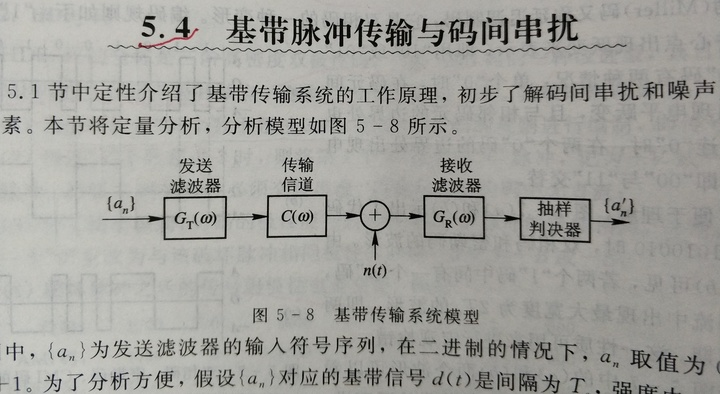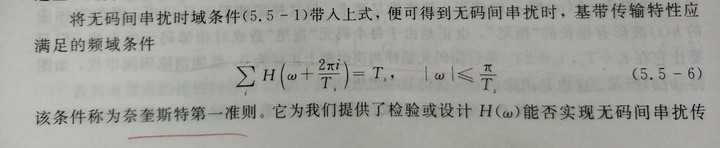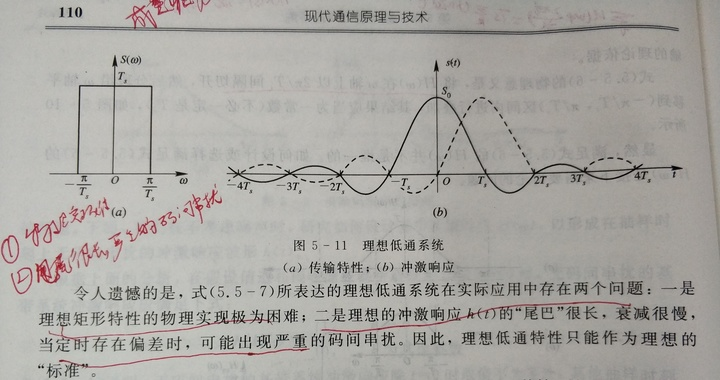%%%%%%%%%%%%%%%%%%%%% rcosdesign函数使用 %%%%%%%%%%%%%%%%%
%%%%%%%%%%%%%%%%%%%%% test_rcosdesign    %%%%%%%%%%%%%%%%%
%%%%%%%%%%%% 日期：2020年8月4日   作者：飞蓬大将军  %%%%%%%%%%%%%%%%%

%%%%%%%%%程序说明
%%% b = rcosdesign(beta,span,sps,shape)
%%% beta：滚降因子
%%% span: 表示截断的符号范围，对滤波器取了几个Ts的长度
%%% sps:每个符号的采样数
%%% shape:可选择'normal'或者'sqrt'
%%% b:1*（sps*span）的行向量，升余弦或余弦滤波器的系数

%****************************  程序主体 ****************%
clear all;
h1 = rcosdesign(0.25,1,6,'sqrt');
fvtool(h1,'Analysis','impulse'); %将脉冲响应可视化

%****************************  仿真结论 ***************%
%%%%%成型滤波器的使用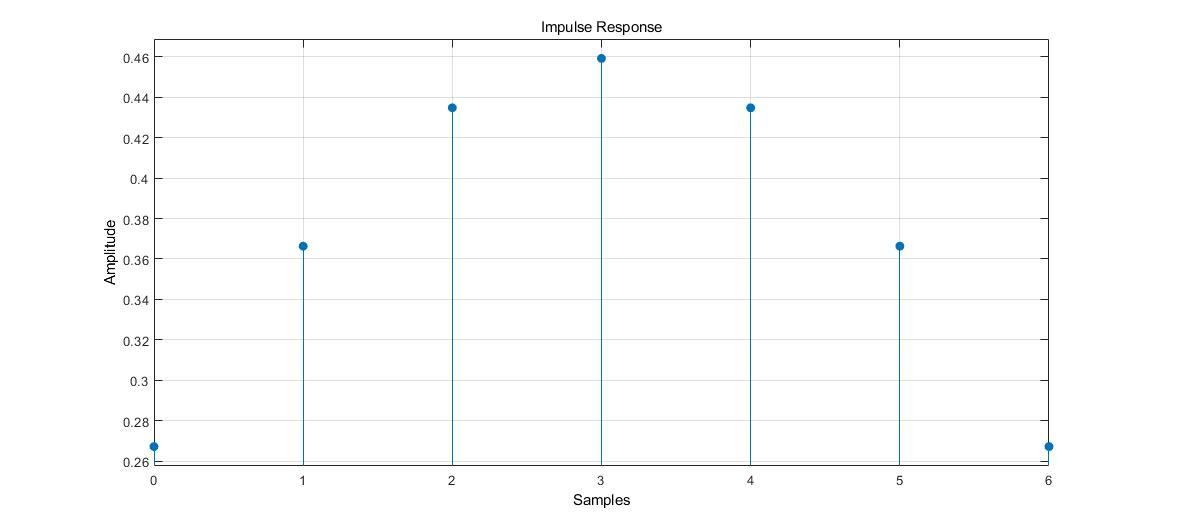%%%%%%%%%%%%%%%%%%%%% 比较不同的滚降成型函数使用 %%%%%%%%%%%%%%%%%
%%%%%%%%%%%%%%%%%%%%%        test_compare_rcos.m    %%%%%%%%%%%%%%%%%
%%%%%%%%%%%%      日期：2020年9月6日   作者：飞蓬大将军  %%%%%%%%%%%%%%%%%

%%%%%%%%%程序说明
%%% b = rcosdesign(beta,span,sps,shape)
%%% beta：滚降因子
%%% span: 表示截断的符号范围，对滤波器取了几个Ts的长度
%%% sps:每个符号的采样数
%%% shape:可选择'normal'或者'sqrt'
%%% b:1*（sps*span）的行向量，升余弦或余弦滤波器的系数

%****************************  程序主体 *************
%%%%%%%%%%%%%%%%%  参数设定   %%%%%%%%%%%%%%
bit_rate = 1000;% 比特率
symbol_rate = 1000;%符号率
sps = 16;%每个符号的采样点数
fc = 2000; %载波频率
fs = 16000; %采样频率

%%%%%%%%%%%%%%%%%   信源   %%%%%%%%%%%%%%%%%%%
%%%%随机信号
source_number = 352;
msg_source = randi([0 1],1,source_number);
%给出标志性的帧头，方便调试

frame_pre = ones(1,32); %用于捕获和同步
frame_begin = [0 1 1 1 1 1 1 0]; %帧开始标志
frame_end = [0 1 1 1 1 1 1 0]; %帧结束标志

%%%%组帧

%%%%%%%%%%%%%%%%%  发射机  %%%%%%%%%%%%%%%%%%%

aaa = 1;
%%%%%双极性变换
bipolar_msg_source = 2*frame_msg-1; %双极性信号

% %波形观察
% figure(1);
% plot(bipolar_msg_source);
% title('时域波形');
% figure(2);
% plot(abs(fft(bipolar_msg_source)));
% title('频域波形');
% t1 = abs(fft(bipolar_msg_source));

%%%上采样
bipolar_msg_source_temp = [bipolar_msg_source',zeros(size(bipolar_msg_source,2),sps-1)];
length_x = size(bipolar_msg_source_temp,1);
length_y = size(bipolar_msg_source_temp,2);
up16_bipolar_msg_source = reshape(bipolar_msg_source_temp',1,length_x * length_y);

% %波形观察
% figure(3);
% plot(up16_bipolar_msg_source);
% title('时域波形');
% figure(4);
% plot(abs(fft(up16_bipolar_msg_source)));
% title('频域波形');
%
% t2 = abs(fft(up16_bipolar_msg_source));

%%%%%%%滤波器
%%%%滚降滤波
%rcos_msg_source1= rcosflt(bipolar_msg_source,1000,16000);
% rolloff_factor默认为0.5

%%%滚降滤波器
rollof_factor = 0.5; %滚降因子,可调整
rcos_fir = rcosdesign(rollof_factor,6,sps);

%%%%滚降滤波
rcos_msg_source1 = filter(rcos_fir,1,up16_bipolar_msg_source);

rcos_msg_source2_temp = conv(up16_bipolar_msg_source,rcos_fir);
filter_start = (length(rcos_fir)-1)/2;
rcos_msg_source2 = rcos_msg_source2_temp(1,filter_start+1:length(rcos_msg_source2_temp)- filter_start);

aaa = 1;
%波形观察
figure(1);
plot(rcos_msg_source1);
title('时域波形');
figure(2);
plot(abs(fft(rcos_msg_source1)));
title('频域波形');

%波形观察
figure(3);
plot(rcos_msg_source2);
title('时域波形');
figure(4);
plot(abs(fft(rcos_msg_source2)));
title('频域波形');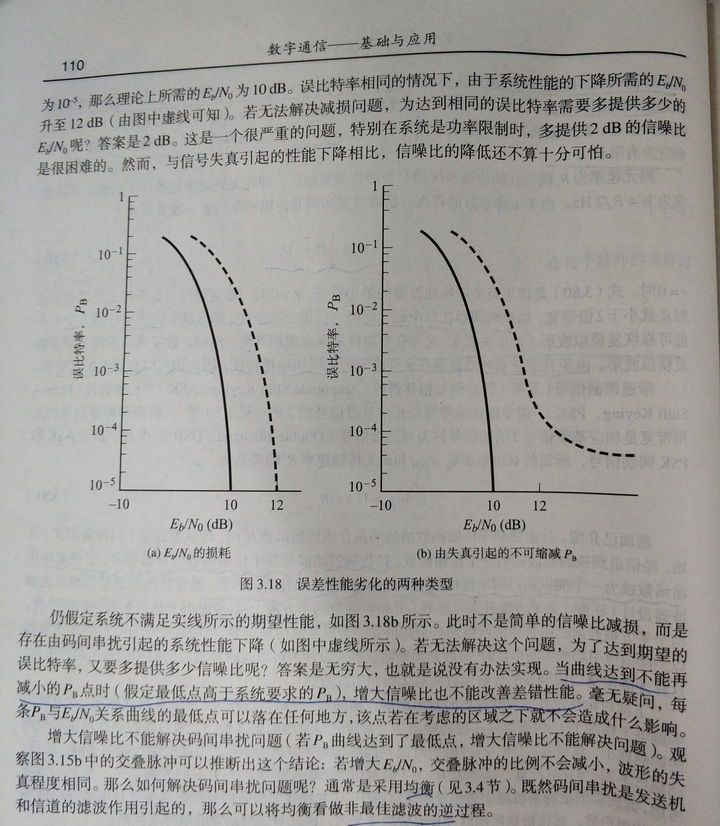（3）匹配滤波器

r ( t ) = s ( t ) + n ( t ) r(t) = s\left( t \right) + n(t)

s ( t ) s\left( t \right) 是数字信号，频谱函数是 S ( ω ) S\left( \omega \right) n ( t ) n(t) 为功率谱密度是 n 0 / 2 {n_0}/2 的高斯白噪声。

H ( ω ) = K S ∗ ( ω ) e − j ω t 0 H\left( \omega \right) = K{S^*}\left( \omega \right){e^{ - j\omega {t_0}}}

h ( t ) = k s ( t 0 − t ) h\left( t \right) = ks\left( {{t_0} - t} \right)

“一方面，从幅频特性来看，匹配滤波器和输入信号的幅频特性完全一样。这也就是说，在信号越强的频率点，滤波器的放大倍数也越大；在信号越弱的频率点，滤波器的放大倍数也越小。这就是信号处理中的“马太效应”。也就是说，匹配滤波器是让信号尽可能通过，而不管噪声的特性。因为匹配滤波器的一个前提是白噪声，也即是噪声的功率谱是平坦的，在各个频率点都一样。因此，这种情况下，让信号尽可能通过，实际上也隐含着尽量减少噪声的通过。这不正是使得输出的信噪比最大吗？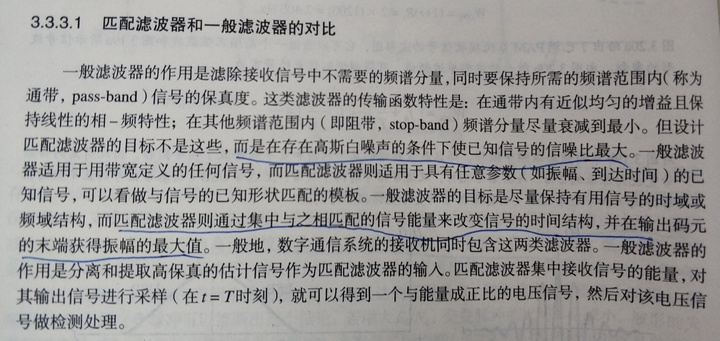（4）最佳采样点的信噪比条件是最好的，因此在最佳采样点进行采样和判决。相比于不在最佳采样点采样和判决，最佳采样点的判决可以使得误码率更接近理论误码率性能。

（5）信噪比与 E b / N 0 {E_b}/{N_0} 有关系吗？ E b / N 0 {E_b}/{N_0} 这个量有单位吗？在什么位置开始定义 E b / N 0 {E_b}/{N_0} 的？为什么数字通信，分析误码率曲线时，横坐标是 E b / N 0 {E_b}/{N_0} 呢？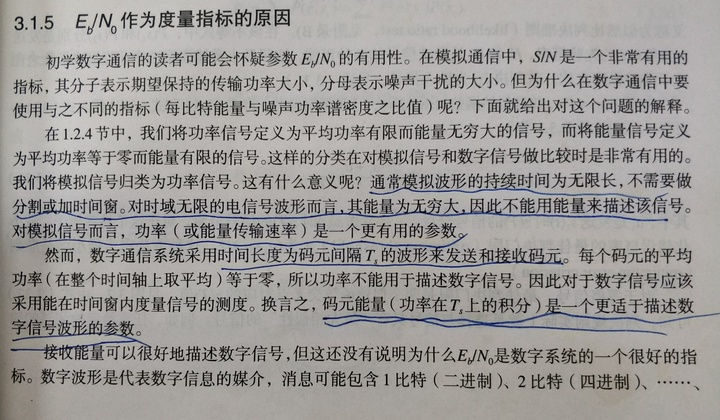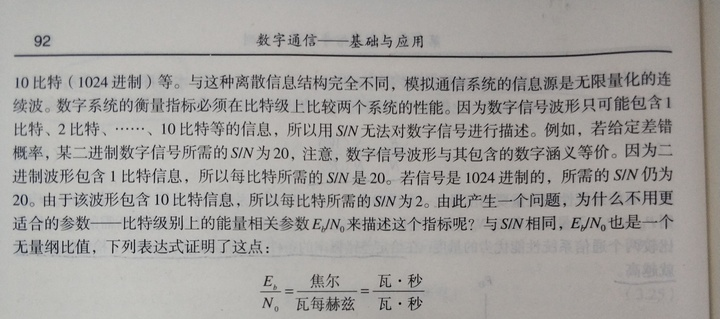E s Es E b {{E_b}} 的关系要联合考虑，编码和调制的影响：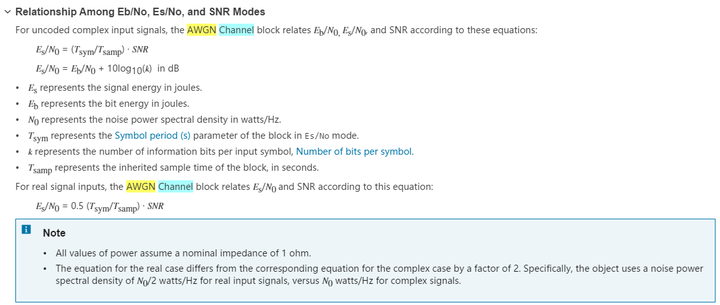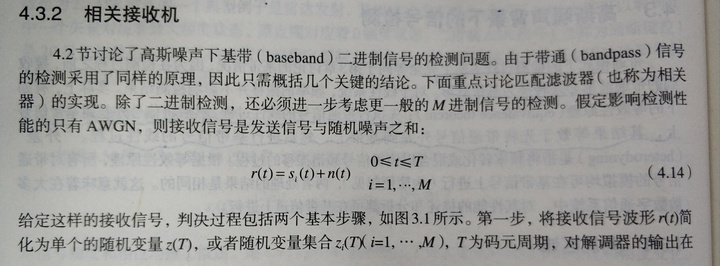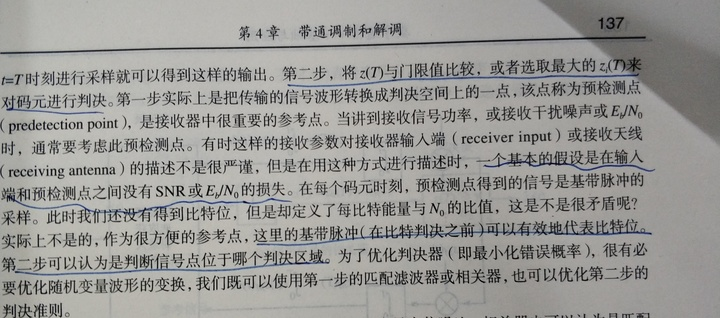（6）高斯白噪声信道时，白噪声怎么加到信号上去呢？

%%%%%%%%%%%%%%%%%%%%%     加噪声函数使用    %%%%%%%%%%%%%%%%%
%%%%%%%%%%%% 日期：2020年8月4日   作者：飞蓬大将军  %%%%%%%%%%%%%%%%%

%%%%%%%%%程序说明
%%%判断两种方式下的加噪声方式是否一致

%*********************  程序主体    ****************%

% N = 1000000;
% x = cos(0:pi/N:6*pi);
% figure (1);
% plot(x);
% y = awgn(x,10,'measured');
% figure (2);
% plot(y);
%
% sigPower  = sum(abs(x.^2)/length(x));
% noisePower = sum(abs((y-x).^2)/length(y));
% SNR_dB = 10*log10(sigPower/noisePower);

%%%%%%%%结论
%%%%计算可知SNR_dB约等于10dB，当信号长度N越长，SNR_dB越接近10dB，因此可认为两种加噪声方式在统计意义上一致的

%%%%%方式一：用awgn函数
x = cos(0:pi/1000000:6*pi);
figure (1);
plot(x);
snr = 10; %单位是dB
y1 = awgn(x,snr,'measured');
figure (2);
plot(y1);

%%%%%方式二：加随机序列
% x = cos(0:pi/1000000:6*pi);
sigPower =  sum(abs(x.^2)/length(x));
noisePower = sigPower /10^(snr/10);
a = sqrt(noisePower);
y2 = x + a*randn(1,length(x));
figure (3);
plot(y2);

%%%%%%%%%%%结论
%%%%两种加噪声方式都可以，按照个人习惯选择即可
%%%%当存在I路、Q路信号时，噪声功率在I路和Q路上是各占一半功率

（7）信号的频域图、眼图怎么看？

%波形观察
figure(1);
plot(rcos_msg_source);
title('时域波形');
figure(2);
plot(abs(fft(rcos_msg_source)));
title('频域波形');

eyediagram(rcos_msg_source(49:end),sps);
title('眼图');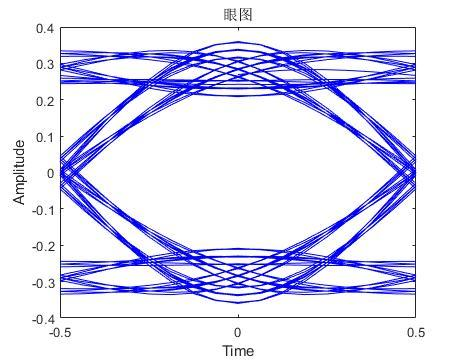五、完整可运行代码附上：

%%%%%%%%%%%%%%%%%%%%%  BPSK调制解调器仿真 %%%%%%%%%
%%%%%%%%%%%%%%%%%%%%%  BPSK_modem_sim1_1_13.m  %%%%%%%%%
%%%%% data:2020年6月10日  原创：算法工匠    改动:飞蓬大将军 %%%%%%%%%%

%%%%%程序说明
%%完成了BPSK调制解调仿真,比较不同信噪比下的误码率性能
%通信体制具体内容如下：
%调制方式：BPSK   编码方式：无
%滚降因子：0.5
%解调方式：相干解调  译码方式：无
%噪声：线性高斯白噪声

%%%%    仿真环境
%软件版本：MATLAB R2019a

%%%    sim系列说明
%1_7：明确了Es/N0(dB)=10log10(0.5Tsym/Tsamp)+SNR(dB) for real input signals

clear all;
close all;
format long;
tic;

%********************** 程序主体 ************%

%%%%%%%%%%%%%%%%%  参数设定   %%%%%%%%%%%%%%
bit_rate =1000;% 比特率
symbol_rate = 1000;%符号率
sps =16;%每个符号的采样点数
fc =2000; %载波频率
fs =16000; %采样频率

%%%%%%%%%%%%%%%%%   信源   %%%%%%%%%%%%%%%%%%%
%%%%随机信号
msg_source = [ones(1,20) zeros(1,20) randi([0 1],1,99960)];
%给出标志性的帧头，方便调试

%%%%%%%%%%%%%%%%%  发射机  %%%%%%%%%%%%%%%%%%%
%%%%%%%%调制器
%%%%%双极性变换
bipolar_msg_source = 2*msg_source-1; %双极性信号

%%%滚降滤波器
rollof_factor = 0.5; %滚降因子,可调整
rcos_fir = rcosdesign(rollof_factor,6,sps);

%%%插值
bipolar_msg_source_temp = [bipolar_msg_source',zeros(size(bipolar_msg_source,2),sps-1)];
length_x = size(bipolar_msg_source_temp,1);
length_y = size(bipolar_msg_source_temp,2);
up16_bipolar_msg_source = reshape(bipolar_msg_source_temp',1,length_x * length_y);

%%%%滚降滤波
rcos_msg_source = filter(rcos_fir,1,up16_bipolar_msg_source);

% %波形观察
% figure(1);
% plot(rcos_msg_source);
% title('时域波形');
% figure(2);
% plot(abs(fft(rcos_msg_source)));
% title('频域波形');
%
% eyediagram(rcos_msg_source(49:end),sps);
% title('眼图');

%%%%%载波发送
time = [1:length(rcos_msg_source)];
rcos_msg_source_carrier = rcos_msg_source.*cos(2*pi*fc.*time/fs);
%%%%%%后续考虑直接用复数载波信号实现，更加方便

% %波形观察
% figure(3);
% plot(rcos_msg_source_carrier);
% title('时域波形');
% figure(4);
% plot(abs(fft(rcos_msg_source_carrier)));
% title('频域波形');

%%%%%%%%%%%%%%%%%  信道    %%%%%%%%%%%%%%%%%%%
%设置信噪比，单位dB
ebn0 =[-6:8];
snr = ebn0 - 10*log10(0.5*16);

for i =1:length(snr)

%%%线性高斯白噪声信道

% %波形观察
% figure(5);
% title('时域波形');
% figure(6);
% title('频域波形');

%%%%%%%%%%%%%%%%%  接收机  %%%%%%%%%%%%%%%%%%%
%%%%%%载波恢复
%%%相干解调

% %波形观察
% figure(7);
% title('时域波形');
% figure(8);
% title('频域波形');

%%%%%%%滤波
%%%%低通滤波
fir_lp =fir1(128,0.2); %截止频率为0.2*(fs/2)
%延迟64个采样点输出

% %波形观察
% figure(9);
% plot(rcos_msg_source_lp);
% title('时域波形');
% figure(10);
% plot(abs(fft(rcos_msg_source_lp)));
% title('频域波形');

%%%%%%匹配滤波
%生成匹配滤波器
rollof_factor =0.5;
rcos_fir = rcosdesign(rollof_factor,6,sps);
%滤波
rcos_msg_source_MF = filter(rcos_fir,1,rcos_msg_source_lp);

% %波形观察
% figure(11);
% plot(rcos_msg_source_MF);
% title('时域波形');
% figure(12);
% plot(abs(fft(rcos_msg_source_MF)));
% title('频域波形');

%%%%%最佳采样
%%%选取最佳采样点
decision_site = 160; %(96+128+96)/2 =160 三个滤波器的延迟 96 128 96

%每个符号选取一个点作为判决
rcos_msg_source_MF_option = rcos_msg_source_MF(decision_site:sps:end);
%涉及到三个滤波器，固含有滤波器延迟累加

%%%判决
msg_source_MF_option_sign= sign(rcos_msg_source_MF_option);

% %波形观察
% figure(13);
% plot(msg_source_MF_option,'-*');
% title('判决结果');
%
% eyediagram(rcos_msg_source,sps);
% title('发射端眼图');
% eyediagram(rcos_msg_source_MF,sps);
% title('接收端眼图');
%
% scatterplot(rcos_msg_source(48+1:16:end-48));
% title('BPSK星座图');

%%%%%%%%%%%%%%%%%   信宿    %%%%%%%%%%%%%%%%%%%%
%%%误码率性能比对
%[err_number,bit_err_ratio]=biterr(x,y)
[err_number(i),bit_err_ratio(i)]=biterr(msg_source(1:length(rcos_msg_source_MF_option)),(msg_source_MF_option_sign+1)/2);

end %for i
toc;
%%%%%%%%%%%%%%%%%   仿真结果    %%%%%%%%%%%%%%%%%%%%
ber = berawgn(ebn0,'psk',2,'nondiff');
semilogy(ebn0,bit_err_ratio,'-*',ebn0,ber,'-+');
xlabel('比特信噪比');
ylabel('误码率');
title('不同信噪比下误码率仿真曲线');
legend('实验曲线','理论曲线');
grid on;
%bit_err_ratio = [0.244582012381114   0.213429208628777   0.187996919722775   0.163494714524307
%0.131351821663950   0.105409486853817   0.081767359062316   0.05948535368183
%0.039983598523867   0.025562300607055   0.014161274514706   0.007020631856867
%0.003290296126651   0.000920082807453   0.000360032402916 ]
%%%%%%%%%%%%%%%%%   结论    %%%%%%%%%%%%%%%%%%%%

%完成了BPSK调制解调器的仿真
%没有包含编译码内容
%2020-6-10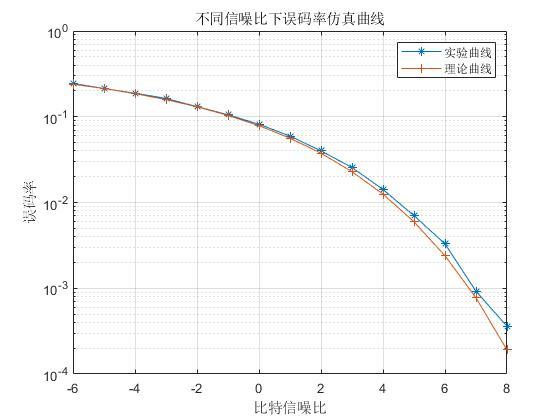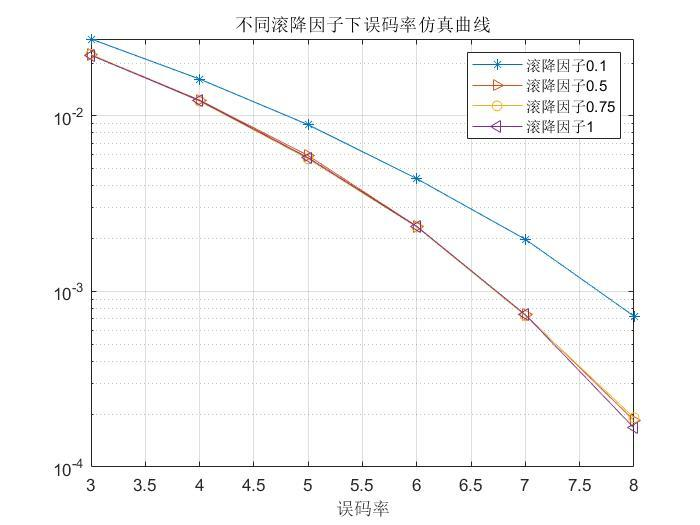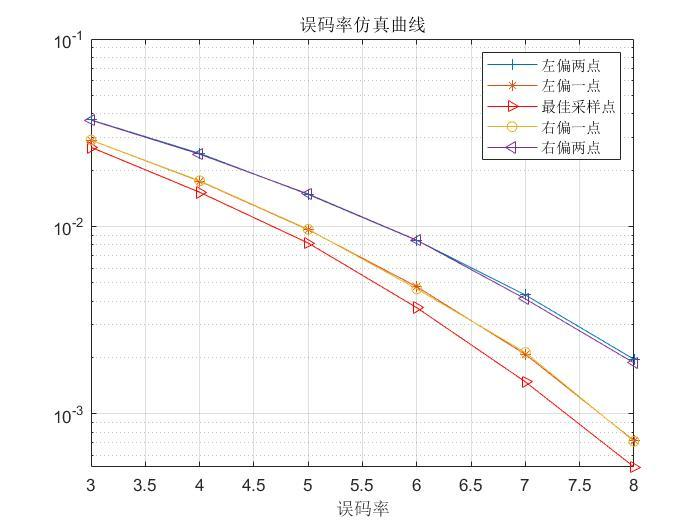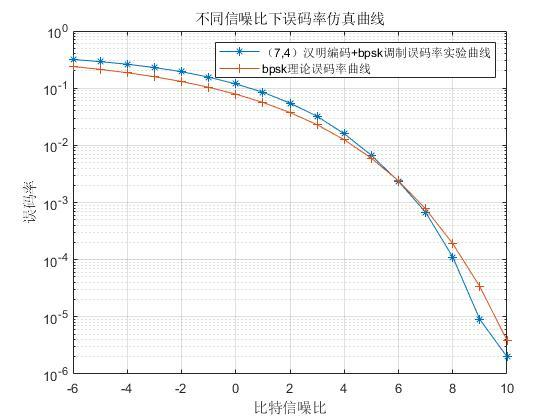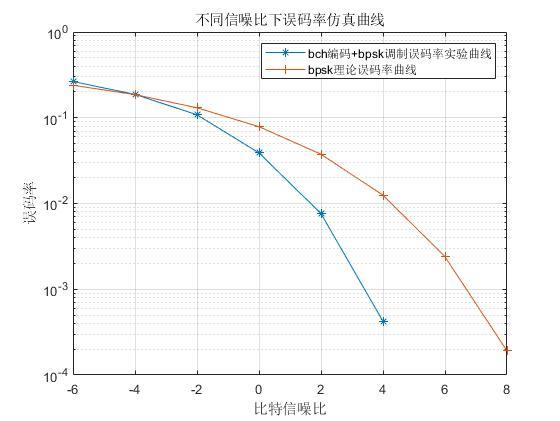10-12
03-13

10-136320
03-243万+
01-272万+
07-291177
11-221078
05-16
03-18135
09-10381
07-15
09-121224
05-201万+
09-202563
11-22370
04-20524
06-073964¥2 ¥4 ¥6 ¥10 ¥20余额支付 (余额：-- )扫码支付获取中扫码支付点击重新获取扫码支付1.余额是钱包充值的虚拟货币，按照1:1的比例进行支付金额的抵扣。
2.余额无法直接购买下载，可以购买VIP、C币套餐、付费专栏及课程。余额充值